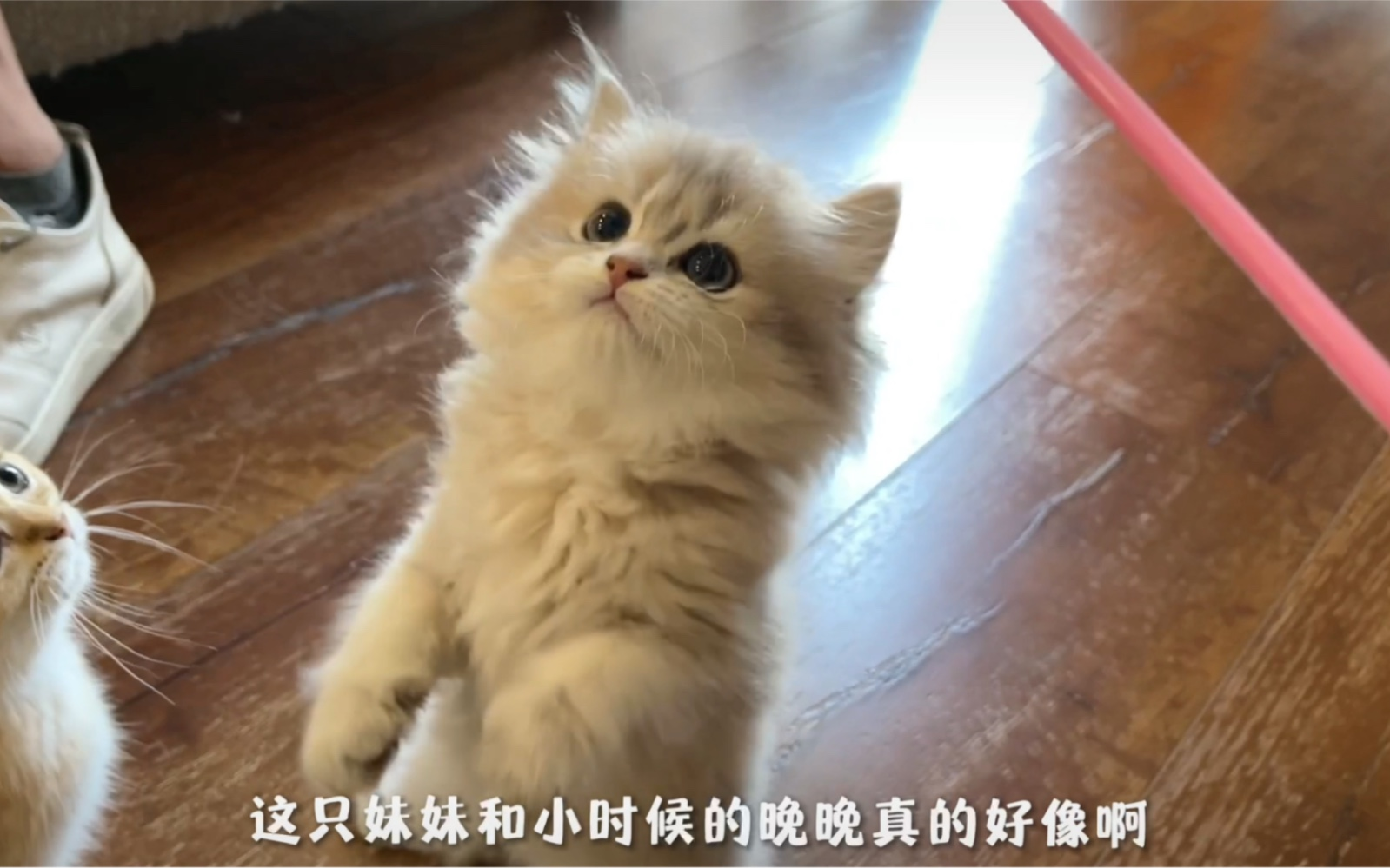# 讨论精选:这就是有了二胎，一胎的反应？（我永远爱晚晚）[一次目更~]

b站评论区果然有大病，人家养几只猫都要管[辣眼睛]

[脱单doge]晚晚永远都是妈妈的心头爱

[OK][OK][OK]

{\__/}

( • . •)

/ >⚪️

{\__/}

( • – •)

⚪️< \

{\__/}

( • . •)

/ >⚪️

{\__/}

( • – •)

⚪️< \

{\__/}

( • . •)

/ >⚪️

{\__/}

( • – •)

⚪️< \

Σ(っ °Д °;)っ

{\__/}

(ಡωಡ)

⚪️< \